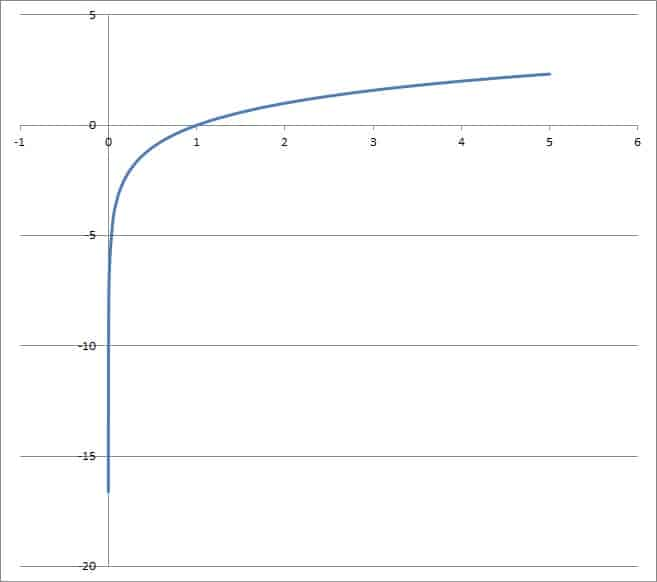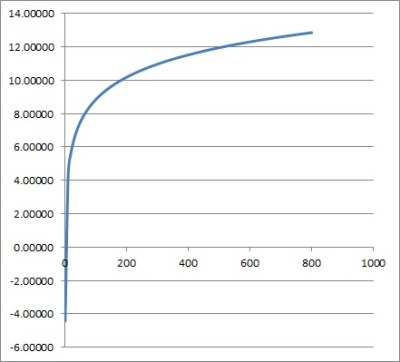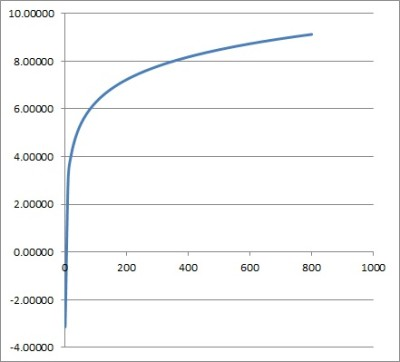# Logarithm Change Of Base (3 Key Things To Know)

A logarithm change of base is useful in many scenarios when you are working with either exponents or logarithms

So, what do you need to know about a logarithm change of base?  The logarithm change of base formula is given by: logb(x) = loga(x) / loga(b), where a, b, and x are positive real numbers and a, b are both not equal to 1. This formula helps us to solve logarithmic equations, simplify expressions, or switch to log bases that a calculator can compute.

Of course, you can switch bases any way you like to make your equation easier to work with.

In this article, we’ll talk about logarithm change of base, how it works, and why it works. We’ll also look at several examples of how to use this concept.

Let’s get started.

## What Is The Logarithm Change Of Base Formula?

The logarithm change of base formula is given by:

• logb(x) = loga(x) / loga(b)

where a, b, and x are positive real numbers, a is not equal to 1, and b is not equal to 1.

### What Does The Change Of Base Formula Do?

The logarithm change of base formula lets you switch from a logarithm with a base of b to a logarithm with a base of a.  The formula works as long as a and b are positive real numbers and both are not equal to 1.The logarithm change of base formula requires the bases a and b to be positive numbers that are not equal to 1. Here we see the graph of f(x) = log2(x).

Remember that the logarithm change of base formula changes a logarithm in one base to a quotient (fraction) of logarithms with another base.

The logarithm change of base formula can be useful in the following situations:

• You want to switch to a common logarithm base (such as 10 or e ~ 2.718).  A calculator usually has buttons for a base of 10 or e, but not for other bases.
• You want to combine terms and simplify by using rules of logarithms.  For example, the rule loga(x) + loga(y) = loga(xy) depends on the base of all the logarithms being the same.
• You want to solve logarithmic equations (we will see examples of this later).
• For x = a, we get the formula logb(a) = loga(a) / loga(b), which simplifies to logb(a) = 1 / loga(b), a sort of “reciprocal rule”.

### How Does Log Change Of Base Work? (Change Of Base Formula Proof)

We will begin by naming the right side of the equation “y” and then proving that y is equal to the left side of the equation.

So, let y = loga(x) / loga(b).  Then:

• y = loga(x) / loga(b)  [by the definition of y that we made]
• yloga(b) = loga(x)  [multiplied by loga(b) on both sides]
• loga(by) = loga(x)  [by logarithm rule of a power: loga(by) = yloga(b)]
• a^(loga(by)) = x  [by formula to convert logarithm to exponential: logB(N) = E is equivalent to BE = N; here, B = a, E = loga(by), and N = x]
• (by) = x  [simplified by rule that a^(loga(N)) = N; here, N = by]
• logb(by) = logb(x)  [take log base b of both sides]
• y = logb(x)  [simplified by rule that logb(by) = y]

Since y = logb(x), this means that logb(x) = loga(x) / loga(b).  This is the logarithm change of base formula.

## How To Change The Base Of A Logarithm

It helps to see concrete examples of how to change the base of a logarithm, so let’s work on some now.

### Example 1: Change The Base Of A Logarithm (Base 2 To Base 10)

Let’s say we want to convert log2(1000) to a base of 10.

We will use the logarithm change of base formula with a = 10, b = 2, and x = 1000:

• logb(x) = loga(x) / loga(b)  [logarithm change of base formula]
• log2(1000) = log10(1000) / log10(2)  [substitute a = 10, b = 2, and x = 1000]
• log2(1000) = log10(103) / log10(2)  [1000 = 103]
• log2(1000) = 3 / log10(2)

So, after changing the base, we see that log2(1000) is equivalent to 3 / log10(2).

### Example 2: Change The Base Of A Logarithm (Base 10 To Base e)

Let’s say we want to convert log10(200) to a base of e (remember that e is approximately 2.718).

We will use the logarithm change of base formula with a = e, b = 10, and x = 200:

• logb(x) = loga(x) / loga(b)  [logarithm change of base formula]
• log10(200) = loge(200) / loge(10)  [substitute a = e, b = 10, and x = 200]
• log10(200) = loge(200) / loge(10)

So, after changing the base, we see that log10(200) is equivalent to loge(200) / loge(10).

If you wish, you can further simplify as follows:

• log10(200) = loge(200) / loge(10)  [from calculation above]
• log10(200) = loge(2*102) / loge(10)  [factor 200 as 2*102]
• log10(200) = (loge(2) + loge(102)) / loge(10)  [log of a product rule: loga(xy) = log­a(x) + loga(y)]
• log10(200) = (loge(2) + 2loge(10)) / loge(10)  [by logarithm rule of a power: loge(102) = 2loge(10)]
• log10(200) = (loge(2) / loge(10)) + (2loge(10) / loge(10))  [split the fraction into two parts]
• log10(200) = (loge(2) / loge(10)) + 2  [loge(10) cancels]

### Example 3: Change The Base Of A Logarithm (Base 5 To Base 10)

Let’s say we want to convert log5(600) to a base of 10.

We will use the logarithm change of base formula with a = 10, b = 5, and x = 600:

• logb(x) = loga(x) / loga(b)  [logarithm change of base formula]
• log5(600) = log10(600) / log10(5)  [substitute a = 10, b = 5, and x = 600]
• log5(600) = log10(6*102) / log10(5)  [600 = 6*102]
• log5(600) = (log10(6) + log10(102)) / log10(5)
• log5(600) = (log10(6) + 2) / log10(5)

So, after changing the base, we see that log5(600) is equivalent to (log10(6) + 2) / log10(5).

### Example 4: Change The Base Of A Logarithm (Base e To Base 2)

Let’s say we want to convert loge(81) to a base of 2.

We will use the logarithm change of base formula with a = 2, b = e, and x = 81:

• logb(x) = loga(x) / loga(b)  [logarithm change of base formula]
• loge(81) = log2(81) / log2(e)  [substitute a = 2, b = e, and x = 81]
• loge(81) = log2(34) / log2(e)  [81 = 34]
• loge(81) = 4log2(3) / log2(e)

So, after changing the base, we see that loge(81) is equivalent to 4log2(3) / log2(e).

## How To Solve A Logarithmic Equation Using Change Of Base Formula

We can also use the logarithm change of base formula to solve logarithmic equations.  Here are some examples.

### Example 1: Solving A Logarithmic Equation Using Change Of Base Formula

Let’s say we want to solve the following logarithmic equation:

• log2(x) + log8(x) = 12

Since the logarithms do not have the same base, we cannot use a rule to combine them.  So, we will use the change of base formula to help us.

We will convert the base 8 to a base of 2.  So, we use the formula with a = 2, b = 8, and x as itself:

• logb(x) = loga(x) / loga(b)  [logarithm change of base formula]
• log8(x) = log2(x) / log2(8)  [substitute a = 2, b = 8, and x = x]
• log8(x) = log2(x) / log2(23)  [8 = 23]
• log8(x) = log2(x) / 3

Now we will make the substitution log8(x) = log2(x) / 3 in the original logarithmic equation:

• log2(x) + log8(x) = 12  [original logarithmic equation]
• log2(x) + log2(x) / 3 = 12  [substitute log8(x) = log2(x) / 3]
• 3*log2(x) + log2(x) = 3*12  [multiply by 3 on both sides to eliminate fraction]
• 3*log2(x) + log2(x) = 36
• 4*log2(x) = 36  [combine like terms]
• log2(x) = 9

The equivalent of the log equation above is x = 29, or x = 512.  We can verify this solution in the original equation:

• log2(x) + log8(x) = 12  [original logarithmic equation]
• log2(512) + log8(512) = 12 (?) [substitute x = 512]
• log2(29) + log8(83) = 12 (?)  [512 = 29 and 512 = 83]
• 9 + 3 = 12 (?)
• 12 = 12

You can see the graph of the function f(x) = log2(x) + log8(x) below.The graph of the function f(x) = log2(x) + log8(x) intersects the horizontal line y = 12 at x = 512.

### Example 2: Solving A Logarithmic Equation Using Change Of Base Formula

Let’s say we want to solve the following logarithmic equation:

• log3(x2) – log9(x) = 6

Since the logarithms do not have the same base, we cannot use a rule to combine them.  So, we will use the change of base formula to help us.

We will convert the base 9 to a base of 3.  So, we use the formula with a = 3, b = 9, and x as itself:

• logb(x) = loga(x) / loga(b)  [logarithm change of base formula]
• log9(x) = log3(x) / log3(9)  [substitute a = 3, b = 9, and x = x]
• log9(x) = log3(x) / log3(32)  [9 = 32]
• log9(x) = log3(x) / 2

Now we will make the substitution log9(x) = log3(x) / 2 in the original logarithmic equation:

• log3(x2) – log9(x) = 6  [original logarithmic equation]
• log3(x2) – log3(x) / 2 = 6  [log9(x) = log3(x) / 2]
• 2*log3(x2) – log3(x) = 2*6  [multiply by 2 on both sides to eliminate fraction]
• 2*log3(x2) – log3(x) = 12
• 2*2*log3(x) – log3(x) = 12  [use log of a power rule]
• 4log3(x) – log3(x) = 12
• 3log3(x) = 12  [combine like terms]
• log3(x) = 4

The equivalent of the log equation above is x = 34, or x = 81.  We can verify this solution in the original equation:

• log3(x2) – log9(x) = 6  [original logarithmic equation]
• log3(812) – log9(81) = 6  [substitute x = 81]
• log3((34)2) – log9(92) = 6  (?)  [81 = 34 and 81 = 92]
• log3(38) – log9(92) = 6  (?)
• 8 – 2 = 6  (?)
• 6 = 6

You can see the graph of the function f(x) = log3(x2) – log9(x) below.The graph of the function f(x) = log3(x2) – log9(x) intersects the horizontal line y = 6 at x = 81.

## Conclusion

Now you know the logarithm change of base formula, where it comes from, and how to use it.

You can learn all about logarithmic functions here.